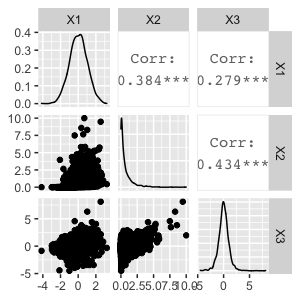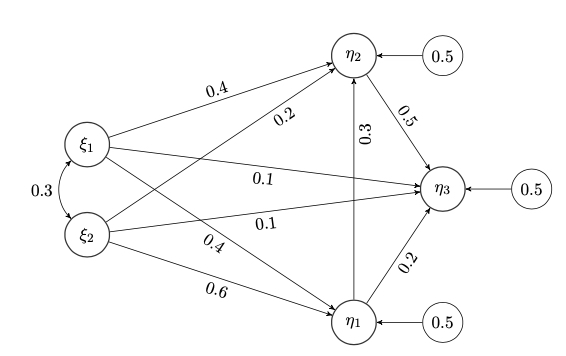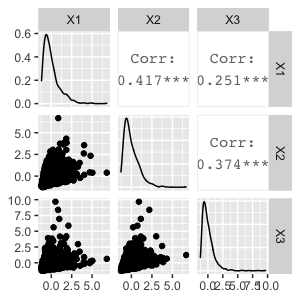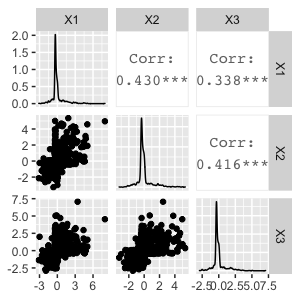# Covsim: Simulating non-normal data with given covariance matrix

#### 2022-04-30

The covsim package includes three methods for simulating non-normal data from distributions with given covariance matrix. The most flexible method is VIne-To-Anything (VITA) (Steffen Grønneberg and Foldnes (2017)), and its implementation and use in covsim is explained in S. Grønneberg, Foldnes, and Marcoulides (2022). VITA allows complete specification of the marginal distributions, and partial specification of bivariate dependencies.

In addition to VITA, two more simple methods are available in covsim, which allows specification of univariate skewness and kurtosis. The independent generator (IG) was proposed by Foldnes and Olsson (2016), while the piecewise linear approach (PLSIM) was proposed by Foldnes and Grønneberg (2021).

# VITA

The function vita() returns a regular vine object as defined by package rvinecopulib (Nagler and Vatter (2019)).
This object may be used to simulate data from the corresponding vine distribution. The function takes a list of marginal distributions and a covariance matrix as essential input. In addition, the user may provide details on the vine structure and the bivariate copulae to be considered at each node in the vine.

## Bivariate case

Let us first consider the bivariate case, where we want a distribution with standard normal marginals coupled by a Clayton copula, whose correlation is $$\rho=.8$$

library("covsim")
mnorm <- list(list(distr = "norm"), list(distr = "norm"))
sigma.target <- matrix(c(1, 0.8, 0.8, 1), 2)
set.seed(1)
calibrated.vita <- vita(mnorm, sigma.target, family_set = "clayton")
#> Tree 1
#>     1 - 2 ( 1 of 1 )
summary(calibrated.vita)
#> $margins #> # A data.frame: 2 x 2 #> margin distr #> 1 norm #> 2 norm #> #>$copula
#> # A data.frame: 1 x 10
#>  tree edge conditioned conditioning var_types  family rotation parameters df
#>     1    1        1, 2                    c,c clayton        0        3.4  0
#>   tau
#>  0.63

The calibrated vita object is made from a Clayton copula with dependence parameter $$\theta=3.4$$. Let us confirm that the vine distribution has correlation $$\rho=.8$$, by simulating a $$n=10^5$$ sample from the vita object:

library("rvinecopulib")
large.sample <- rvine(10^5, calibrated.vita)
cov(large.sample)
#>           [,1]      [,2]
#> [1,] 0.9994719 0.8017954
#> [2,] 0.8017954 0.9985791

## Trivariate case

The motivation for this example is given in S. Grønneberg, Foldnes, and Marcoulides (2022), and we here restrict ourselves to calibration of the vita object. Our target covariance matrix is $\Sigma = \begin{pmatrix} 1 & 0.4 & 0.3 \\ 0.4 & 1 & 0.4 \\ 0.3 & 0.4 & 1 \\ \end{pmatrix},$ and we want the marginals to be the standard normal distribution, a scaled chi-squared distribution with one degree of freedom, and a scaled Student’s $$t$$ distribution with five DFs. The scalings ensure unit variance. In addition, we want to specify the vine structure explicitly, and also the copula family for each bivariate dependency as follows.

sigma.target <- matrix(c(1, 0.4, 0.3, 0.4, 1, 0.4, 0.3, 0.4, 1), 3)
margins <- list(list(distr = "norm"), list(distr = "chisq", df = 1),
list(distr = "t", df = 5))
pcs <- list(list(bicop_dist("clayton"), bicop_dist("joe")),
list(bicop_dist("frank")))
vine_cop <- vinecop_dist(pcs, structure = dvine_structure(1:3))
margin.variances <- c(1, 2, 5/3)
pre <- diag(sqrt(margin.variances/diag(sigma.target)))
vita.target <- pre %*% sigma.target %*% pre
set.seed(1)
calibrated.vita <- vita(margins, vita.target, vc = vine_cop,
verbose = TRUE)
#> Tree 1
#>     1 - 2 ( 1 of 3 )
#>     2 - 3 ( 2 of 3 )
#> Tree 2
#>     1 - 3 ( 3 of 3 )
post <- diag(1/diag(pre))
vita.sample <- rvine(10^5, calibrated.vita) %*% post
round(cov(vita.sample) - sigma.target, 3)
#>        [,1]   [,2]   [,3]
#> [1,] -0.001  0.001 -0.004
#> [2,]  0.001  0.001 -0.002
#> [3,] -0.004 -0.002  0.002
library(GGally)
GGally::ggpairs(data.frame(head(vita.sample, 2*10^3)))Note that if the vc option is not included in the vita() call, the default will be the simple structure given by a d-vine. The copula families in each node (each bivariate association) may be specified by option family_set, where the default ensures that first a Clayton copula is tentatively calibrated. If unsuccesful, next a gauss, joe, gumbel or frank copula are calibrated, in that order. If none of these families can produce the required covariance, vita() returns an error message indicating that no vine distributional class can match the given marginals and covariance matrix.

## SEM example in 20 dimensionsSEM population model, from Li (2016)

sem.pop <- 'Ksi1 =~ start(.8)*x1 +start(.7)*x2 +start(.6)*x3 +start(.5)*x4
Ksi2 =~ start(.8)*x5 + start(.7)*x6 + start(.6)*x7 +start(.5)*x8
Eta1 =~ start(.8)*y1 + start(.7)*y2 + start(.6)*y3 +start(.5)*y4
Eta2 =~ start(.8)*y5 + start(.7)*y6 + start(.6)*y7 +start(.5)*y8
Eta3 =~ start(.8)*y9 + start(.7)*y10 + start(.6)*y11 +start(.5)*y12
Eta1 ~ start(.4)*Ksi1 + start(.6)*Ksi2
Eta2 ~ start(.4)*Ksi1 + start(.2)*Ksi2 + start(.3)*Eta1
Eta3 ~ start(.1)*Ksi1 + start(.1)*Ksi2 + start(.2)*Eta1 +start(.5)*Eta2
Ksi1 ~~ start(.3)*Ksi2; Eta1 ~~ start(.5)*Eta1;
Eta2 ~~ start(.5)*Eta2; Eta3 ~~ start(.5)*Eta3
x1 ~~ start(.5)*x1; x2 ~~ start(.5)*x2
x3 ~~ start(.5)*x3; x4 ~~ start(.5)*x4; x5 ~~ start(.5)*x5
x6 ~~ start(.5)*x6; x7 ~~ start(.5)*x7; x8 ~~ start(.5)*x8
y1 ~~ start(.5)*y1; y2 ~~ start(.5)*y2; y3 ~~ start(.5)*y3
y4 ~~ start(.5)*y4; y5 ~~ start(.5)*y5; y6 ~~ start(.5)*y6
y7 ~~ start(.5)*y7; y8 ~~ start(.5)*y8; y9 ~~ start(.5)*y9
y10 ~~ start(.5)*y10; y11 ~~ start(.5)*y11; y12 ~~ start(.5)*y12'
library(lavaan)
sigma.target <- lavInspect(sem(sem.pop, data = NULL), "sigma.hat")

Next, we fit a VITA distribution with normal marginals to the target covariance matrix. This is a variant of a data generating distribution used in the simulation study of Foldnes and Grønneberg (2021). First, the margins are scaled to match the target variances. Then, we calibrate a VITA distribution. Note that we do not specify which family of copulae to use, so the default Clayton copula is used. Finally, a list of 1000 samples, each of sample size 1000, is drawn from the calibrated vita distribution.

marginsnorm <- lapply(X = sqrt(diag(sigma.target)),
function(X) list(distr = "norm", sd = sqrt(X)))
randomsamples <- replicate(10^3, rvine(10^3, vitadist))

The above calibration step is time-consuming, since it is high-dimensional. With 20 variables, the calibration step required 1.8 hours (again using a 2.3 GHz 8-Core Intel Core i9 CPU). This step is only performed once. When completed, random samples can be drawn at a relatively fast rate. Producing 1000 samples each of size 1000 took one minute to complete. Finally, we note that the calibration step may be performed faster by specifying option Nmax=$$10^5$$ when calling , at the expense of reduced precision in covariance matching.

## Ordinal-categorical data

We assume that the underlying correlation in a continuous bivariate distribution with standard normal marginals is $$\rho=0.5$$, and we discretize into three categories using thresholds $$\tau_1=0$$ and $_2=1$. This means that we consider simulated data of the form [ X_i = { $\begin{matrix} 1, & \text{if } \xi_i \leq \tau_1 \\ 2, & \text{if } \tau_1 < \xi_i \leq \tau_2 \\ 3, & \text{if } \xi_i > \tau_2 \\ \end{matrix}$ . = { $\begin{matrix} 1, & \text{if } \xi_i \leq 0 \\ 2, & \text{if } 0 < \xi_i \leq 1 \\ 3, & \text{if } \xi_i > 1 \\ \end{matrix}$

. ] for $$i=1,2$$, where $$(\xi_1,\xi_2)$$ is a continuous random vector simulated using VITA. Both ordinal variables have proportions $$0.5, 0.34,$$ and $$0.16$$. We inquire whether the polychoric correlation estimator used in ordinal SEM becomes biased when we replace the bivariate normal with a Clayton or a Joe copula. So first, we determine parameters for the latter two copulas such that, when marginals are standard normal, the Pearson correlation is $$0.5$$. We then discretize a large sample from each of the VITA distributions and estimate the polychoric correlation. The estimates turn out to be very biased:

sigma.target <- matrix(c(1, 0.5, 0.5, 1), 2)
set.seed(1)
vita_clayton <- vita(list(list(distr = "norm"), list(distr = "norm")),
sigma.target, family_set = "clayton")
#> Tree 1
#>     1 - 2 ( 1 of 1 )
set.seed(1)
vita_joe <- vita(list(list(distr = "norm"), list(distr = "norm")),
sigma.target, family_set = "joe")
#> Tree 1
#>     1 - 2 ( 1 of 1 )
clayton.disc <- apply(rvine(10^5, vita_clayton), 2, cut,
breaks = c(-Inf, 0, 1, Inf), labels = FALSE)
joe.disc <- apply(rvine(10^5, vita_joe), 2, cut,
breaks = c(-Inf, 0, 1, Inf), labels = FALSE)
library(psych)
#polychoric correlation (based on underlying normality) is severely biased (downards for Clayton, and upwards for Joe)
polychoric(clayton.disc)$rho #> [,1] [,2] #> [1,] 1.000000 0.419161 #> [2,] 0.419161 1.000000 polychoric(joe.disc)$rho
#>           [,1]      [,2]
#> [1,] 1.0000000 0.5918562
#> [2,] 0.5918562 1.0000000

# IG and Piecewise linear methods

The IG and PLSIM methods require just specifying the kurtosis and skewness of each marginal distribution, together with the covariance matrix. Hence, the marginals are not fully controlled, in contrast to VITA.

Let us require skewness=2 and excess kurtosis =7 in each of three marginal distributions. The covariance matrix is specified as $\Sigma = \begin{pmatrix} 1 & 0.4 & 0.3 \\ 0.4 & 1 & 0.4 \\ 0.3 & 0.4 & 1 \\ \end{pmatrix},$

## IG

Function rIG() returns a list of simulated samples, of length specified by option reps. The default is reps=1 (one sample only), so we need to append []:

skewness=rep(2, 3)
excesskurtosis=rep(7,3)
sigma.target <- matrix(c(1, 0.4, 0.3, 0.4, 1, 0.4, 0.3, 0.4, 1), 3)
set.seed(1)
ig.sample <- rIG(10^4, sigma.target, skewness, excesskurtosis )[]
round(cov(ig.sample)-sigma.target,3)
#>        [,1]   [,2]   [,3]
#> [1,] -0.068 -0.025  0.001
#> [2,] -0.025 -0.035 -0.002
#> [3,]  0.001 -0.002  0.021
psych::skew(ig.sample)
#>  1.710757 1.926682 2.172702
psych::kurtosi(ig.sample)
#>  4.687017 6.328181 8.787962

## PLSIM

The piecewise linear approach is run similarly:

set.seed(1)
pl.sample <- rPLSIM(10^4, sigma.target, skewness, excesskurtosis )[]
pl.sample <- data.frame(pl.sample)
round(cov(pl.sample)-sigma.target,3)
#>       X1     X2     X3
#> X1 0.027  0.015  0.008
#> X2 0.015  0.028 -0.001
#> X3 0.008 -0.001 -0.021
psych::skew(pl.sample)
#>  2.033319 1.891539 1.870833
psych::kurtosi(pl.sample)
#>       X1       X2       X3
#> 7.078430 6.130854 6.264656

Note that rPLSIM() is slower that rIG(), since it requires numerical optimization for each pair of variables. The distributions stemming from PLSIM are different from the IG distributions, even if the covariance matrix and the marginal skewness and kurtosi are identical.

GGally::ggpairs(data.frame(ig.sample)[2:10^3,])GGally::ggpairs(data.frame(pl.sample)[2:10^3,])